# OpenCV 萌新福音：易上手的数字识别实践案例## 一、案例介绍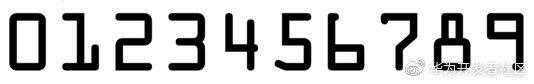​要求：识别出信用卡上的数字，并将其直接打印在原图片上。虽然看起来很蠢，但既然可以将数字打印在图片上，说明已经成功识别数字，因此也可以将其转换为数字文本保存。车牌号识别等项目的思路与此案例类似。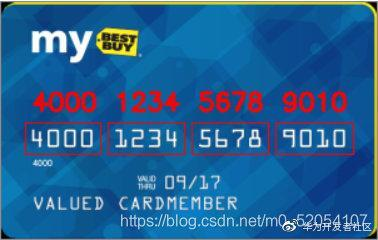## ​二、步骤

1.模板读入

2.模板预处理，将模板数字分开，并排序

3.输入图像预处理，将图像中的数字部分提取出来

4.将数字与模板数字进行匹配，匹配率最高的即为对应数字。

1、模板读入，以及一些包的导入，函数定义等

import cv2 as cvimport numpy as npimport myutilsdef cv_show(name, img):               # 自定义的展示函数    cv.imshow(name, img)    cv.waitKey(0)# 读入模板图n = 'text'img = cv.imread("images/ocr_a_reference.png")# cv_show(n, template)         # 自定义的展示函数，方便显示图片

​2、模板预处理，将模板数字分开，并排序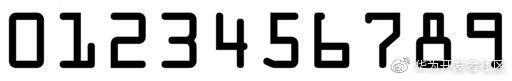​模板的预处理顺序：灰度图，二值化，再进行轮廓检测。需要注意的是 openCV 检测轮廓时是检测白色边框，因此要将模板图的数字二值化变为白色。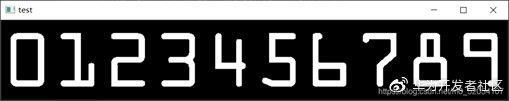# 模板转换为灰度图ref = cv.cvtColor(img, cv.COLOR_BGR2GRAY)# cv_show(n, ref) # 转换为二值图,把数字部分变为白色ref = cv.threshold(ref, 10, 255, cv.THRESH_BINARY_INV)  # 骚写法，函数多个返回值为元组，这里取第二个返回值cv_show(n, ref) # 对模板进行轮廓检测，得到轮廓信息refCnts, hierarchy = cv.findContours(ref.copy(), cv.RETR_EXTERNAL, cv.CHAIN_APPROX_NONE)cv.drawContours(img, refCnts, -1, (0, 0, 255), 2)  # 第一个参数为目标图像# cv_show(n, img)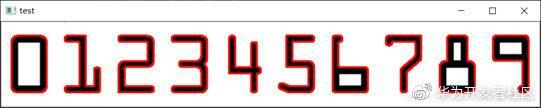​红色部分即为检测出的轮廓。

# 轮廓排序refCnts = myutils.sort_contours(refCnts)digits = {} # 单个轮廓提取到字典中for (i, c) in enumerate(refCnts):    (x, y, w, h) = cv.boundingRect(c)    roi = ref[y:y + h, x:x + w]  # 在模板中复制出轮廓    roi = cv.resize(roi, (57, 88))  # 改成相同大小的轮廓    digits[i] = roi  # 此时字典键对应的轮廓即为对应数字。如键‘1’对应轮廓‘1’

​至此，模板图处理完毕。

3、输入图像预处理，将图像中的数字部分提取出来​在此步骤中需要将信用卡上的每个数字提取出来，并与上一步得到的模板一一匹配。首先初始化卷积核，方便之后 tophat 操作以及闭运算操作使用。

# 初始化卷积核rectKernel = cv.getStructuringElement(cv.MORPH_RECT, (9, 3))sqKernel = cv.getStructuringElement(cv.MORPH_RECT, (5, 5))

​接下来读入图片，调整图片大小，转换为灰度图。

# 待分析图片读入,预处理card_image = cv.imread("images/credit_card_01.png")# cv_show('a', card_image)card_image = myutils.resize(card_image, width=300)   # 更改图片大小gray = cv.cvtColor(card_image, cv.COLOR_BGR2GRAY)# cv_show('gray', gray)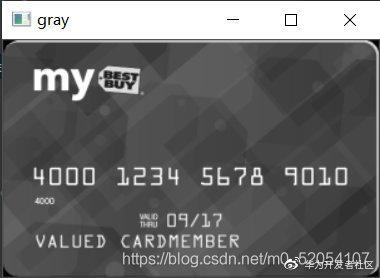​然后进行 tophat 操作，tophat 可以突出图片中明亮的区域，过滤掉较暗的部分：

tophat = cv.morphologyEx(gray, cv.MORPH_TOPHAT, rectKernel)# cv_show('tophat', tophat)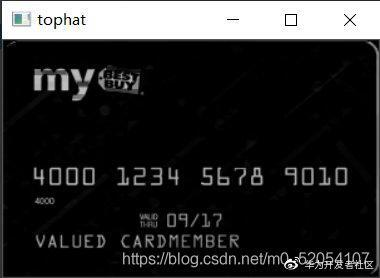​再通过 sobel 算子检测边缘，进行一次闭操作，二值化，再进行一次闭操作，填补空洞。

# x方向的Sobel算子gradX = cv.Sobel(tophat, cv.CV_32F, 1, 0, ksize=3)  gradX = np.absolute(gradX)  # absolute: 计算绝对值min_Val, max_val = np.min(gradX), np.max(gradX)gradX = (255 * (gradX - min_Val) / (max_val - min_Val))gradX = gradX.astype("uint8") # 通过闭操作（先膨胀，再腐蚀）将数字连在一起.  将本是4个数字的4个框膨胀成1个框,就腐蚀不掉了gradX = cv.morphologyEx(gradX, cv.MORPH_CLOSE, rectKernel)# cv_show('close1', gradX) # 二值化thresh = cv.threshold(gradX, 0, 255, cv.THRESH_BINARY | cv.THRESH_OTSU) # 闭操作,填补空洞thresh = cv.morphologyEx(thresh, cv.MORPH_CLOSE, sqKernel)# cv_show('close2', thresh)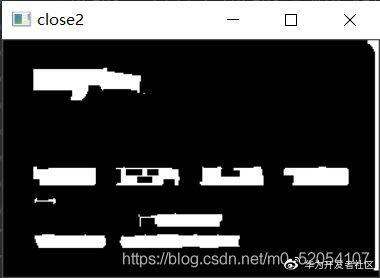​之后就可以查找轮廓了。

threshCnts = cv.findContours(thresh.copy(), cv.RETR_EXTERNAL, cv.CHAIN_APPROX_SIMPLE)card_copy = card_image.copy()cv.drawContours(card_copy, threshCnts, -1, (0, 0, 255), 2)cv_show('Input_Contours', card_copy)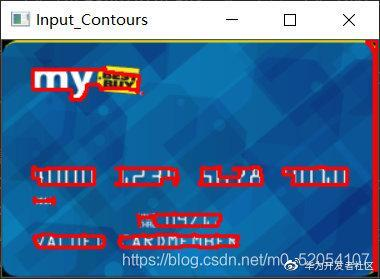​4、模板匹配

locs = []  # 存符合条件的轮廓for i, c in enumerate(threshCnts):    # 计算矩形    x, y, w, h = cv.boundingRect(c)     ar = w / float(h)    # 选择合适的区域，根据实际任务来，这里的基本都是四个数字一组    if 2.5 < ar < 4.0:        if (40 < w < 55) and (10 < h < 20):            # 符合的留下来            locs.append((x, y, w, h)) # 将符合的轮廓从左到右排序locs = sorted(locs, key=lambda x: x)

output = []  # 存正确的数字for (i, (gx, gy, gw, gh)) in enumerate(locs):  # 遍历每一组大轮廓(包含4个数字)    groupOutput = []     # 根据坐标提取每一个组(4个值)    group = gray[gy - 5:gy + gh + 5, gx - 5:gx + gw + 5]  # 往外扩一点    # cv_show('group_' + str(i), group)    # 预处理    group = cv.threshold(group, 0, 255, cv.THRESH_BINARY | cv.THRESH_OTSU)  # 二值化的group    # cv_show('group_'+str(i),group)    # 计算每一组的轮廓 这样就分成4个小轮廓了    digitCnts = cv.findContours(group.copy(), cv.RETR_EXTERNAL, cv.CHAIN_APPROX_SIMPLE)    # 排序    digitCnts = myutils.sort_contours(digitCnts, method="left-to-right") # 计算并匹配每一组中的每一个数值    for c in digitCnts:  # c表示每个小轮廓的终点坐标        z = 0        # 找到当前数值的轮廓,resize成合适的的大小        (x, y, w, h) = cv.boundingRect(c)  # 外接矩形        roi = group[y:y + h, x:x + w]  # 在原图中取出小轮廓覆盖区域,即数字        roi = cv.resize(roi, (57, 88))        # cv_show("roi_"+str(z),roi)         # 计算匹配得分: 0得分多少,1得分多少...        scores = []  # 单次循环中,scores存的是一个数值 匹配 10个模板数值的最大得分         # 在模板中计算每一个得分        # digits的digit正好是数值0,1,...,9;digitROI是每个数值的特征表示        for (digit, digitROI) in digits.items():            # 进行模板匹配, res是结果矩阵            res = cv.matchTemplate(roi, digitROI, cv.TM_CCOEFF)  # 此时roi是X digitROI是0 依次是1,2.. 匹配10次,看模板最高得分多少            Max_score = cv.minMaxLoc(res)  # 返回4个,取第二个最大值Maxscore            scores.append(Max_score)  # 10个最大值        # print("scores：",scores)        # 得到最合适的数字        groupOutput.append(str(np.argmax(scores)))  # 返回的是输入列表中最大值的位置        z = z + 1# 画出来    cv.rectangle(card_image, (gx - 5, gy - 5), (gx + gw + 5, gy + gh + 5), (0, 0, 255), 1)  # 左上角,右下角# putText参数：图片,添加的文字,左上角坐标,字体,字体大小,颜色,字体粗细    cv.putText(card_image, "".join(groupOutput), (gx, gy - 15), cv.FONT_HERSHEY_SIMPLEX, 0.65, (0, 0, 255), 2)

​最后将其打印出来，任务就完成了。

cv.imshow("Output_image_"+str(i), card_image)cv.waitKey(0)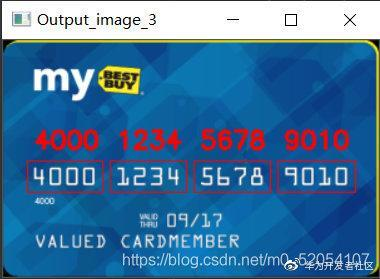## 总结## 评论